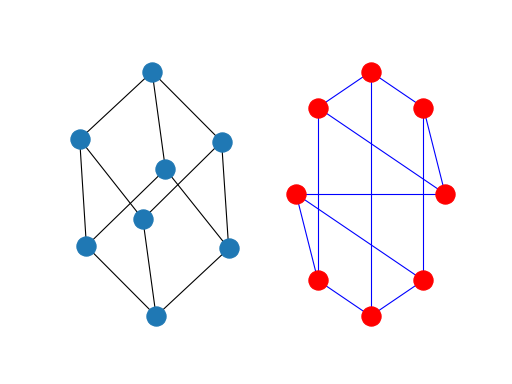# 介绍¶

networkx的结构可以由其源代码的组织看到。该包提供了图形对象类、用于创建标准图形的生成器、用于读取现有数据集的IO例程、用于分析生成的网络的算法和一些基本绘图工具。

## NETWorkX基础¶

>>> import networkx as nx


Graph

DiGraph

MultiGraph

MultiDiGraph

>>> G = nx.Graph()
>>> G = nx.DiGraph()
>>> G = nx.MultiGraph()
>>> G = nx.MultiDiGraph()


## 图¶

• 定向：边缘 定向的 ？边缘对$$（u，v）$$的顺序重要吗？有向图由类名中的“di”前缀指定，例如 DiGraph() . 我们之所以有这样的区别，是因为对于有向图，许多经典的图属性都有不同的定义。

• 多边缘：每对节点之间允许多个边缘吗？正如您可能想象的那样，多个边缘需要不同的数据结构，尽管聪明的用户可以设计边缘数据属性来支持此功能。我们使用前缀“multi”为这类图形提供了标准的数据结构和接口，例如， MultiGraph() .

## 图形创建¶

• 图形生成器——创建网络拓扑的标准算法。

• 从预先存在的（通常是文件）源导入数据。

• 显式添加边和节点。

>>> import networkx as nx
>>> G = nx.Graph()
>>> G.add_edge(1, 2)  # default edge data=1
>>> G.add_edge(2, 3, weight=0.9)  # specify edge data


>>> import math
>>> G.add_node(math.cos)  # any hashable can be a node


>>> elist = [(1, 2), (2, 3), (1, 4), (4, 2)]
>>> elist = [('a', 'b', 5.0), ('b', 'c', 3.0), ('a', 'c', 1.0), ('c', 'd', 7.3)]


## 算法¶

NetworkX提供了许多图形算法。其中包括最短路径和广度优先搜索（参见 traversal ）聚类和同构算法等。还有很多我们还没有开发出来。如果您实现的图形算法可能对其他人有用，请通过 NetworkX Google group 或是吉特鲁布 Developer Zone .

>>> G = nx.Graph()
>>> e = [('a', 'b', 0.3), ('b', 'c', 0.9), ('a', 'c', 0.5), ('c', 'd', 1.2)]
>>> print(nx.dijkstra_path(G, 'a', 'd'))
['a', 'c', 'd']


## 绘图¶

>>> import matplotlib.pyplot as plt
>>> G = nx.cubical_graph()
>>> plt.subplot(121)
<matplotlib.axes._subplots.AxesSubplot object at ...>
>>> nx.draw(G)   # default spring_layout
>>> plt.subplot(122)
<matplotlib.axes._subplots.AxesSubplot object at ...>
>>> nx.draw(G, pos=nx.circular_layout(G), node_color='r', edge_color='b')


(png, hires.png, pdf)examples 更多的想法。

## 数据结构¶

NetworkX使用“字典字典字典”作为基本的网络数据结构。这允许快速查找大型稀疏网络的合理存储。关键是节点，所以 G[u] 返回由边缘属性字典的邻居键控的相邻字典。dict-like对象提供了相邻数据结构的视图。 G.adj 例如 for node, nbrsdict in G.adj.items(): . 表达式 G[u][v] 返回边缘属性字典本身。列表字典也是可能的，但不允许快速边缘检测，也不方便存储边缘数据。

dict的优点dicts的数据结构：

• 使用两个字典查找查找查找边并删除边。

• 更喜欢“列表”，因为使用稀疏存储进行快速查找。

• 更喜欢“设置”，因为数据可以附加到边缘。

• G[u][v] 返回边缘属性字典。

• n in G 节点测试 n 在图中 G .

• for n in G: 循环访问图表。

• for nbr in G[n]: 循环访问邻居。

>>> G = nx.Graph()
{'A': {'B': {}}, 'B': {'A': {}, 'C': {}}, 'C': {'B': {}}}


>>> G = nx.Graph()
>>> G.add_edge(1, 2, color='red', weight=0.84, size=300)
>>> print(G['size'])
300
>>> print(G.edges[1, 2]['color'])
red


1

“一路往下都是字典。”